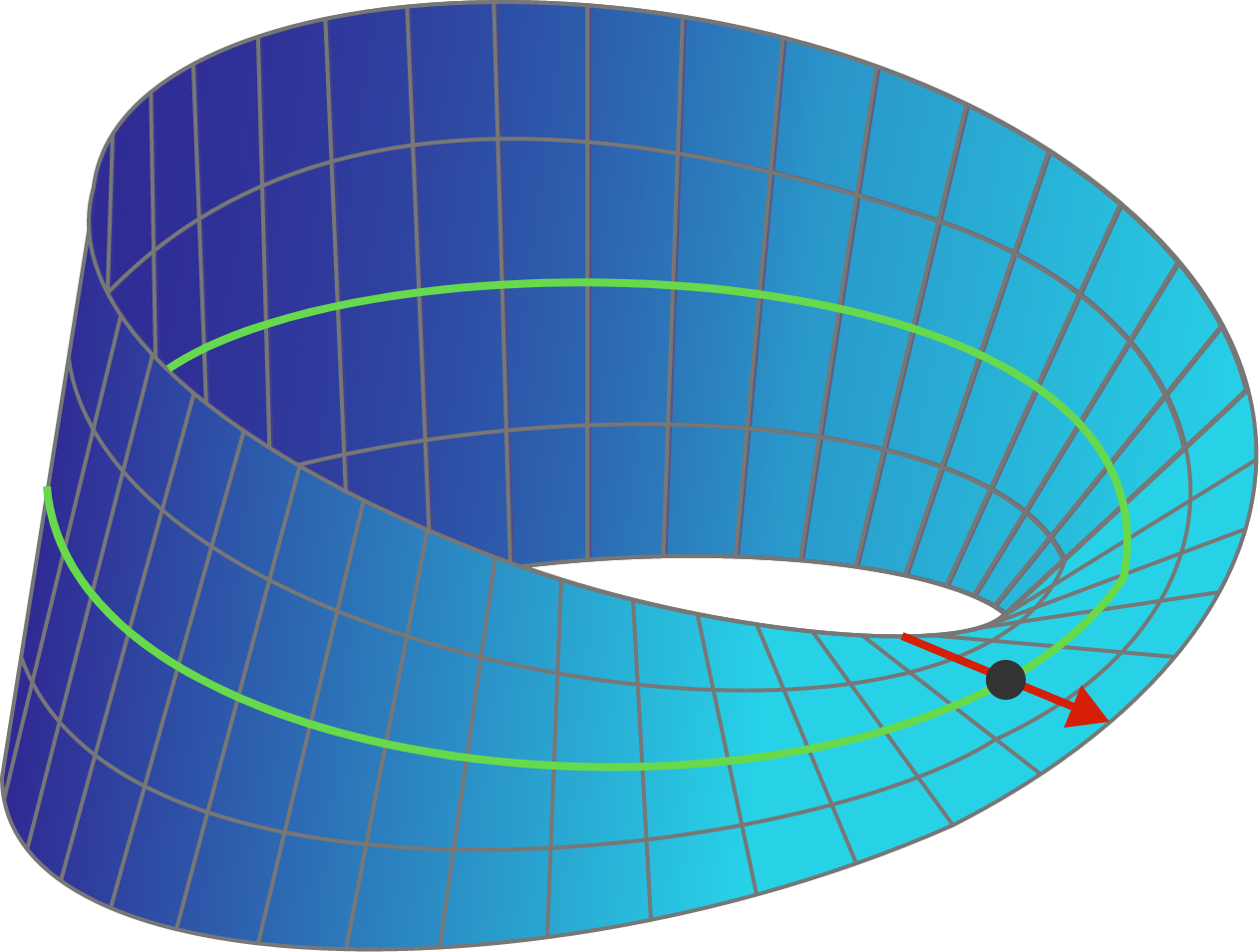Geometry Level 4

Consider a Mobius strip whose width (the red line) is extended indefinitely in both directions throughout 3D space. Note that the Mobius surface will intersect itself.Consider all the regions that this surface would divide 3D space into. How many (disconnected) regions are there? And how many of them have an infinite volume?

Enter your answer as $a . b$, where $a$ is the number of disconnected regions, and $b$ is the number of regions which have infinite volume. For example, if you believe there are 4 regions, of which 3 have infinite volume, enter your answer as $4.3$.

Formally, the Mobius surface as shown in the graphic above is defined as a ruled surface, with a circle as the directrix curve (green), through each point on which (big black point) passes a line (red) perpendicular to the tangent of the circle at that point. As one progresses around the directrix circle, the angle that line makes with the plane of the directrix circle varies by exactly half of the angle around the directrix circle, so that after it has gone around once, the arrow points in the opposite direction, and going around one more time brings the arrow back to the original orientation. We are then extending the red line indefinitely in both directions.

×